# Conditions On Roots Of Quadratics

Let  $$f\left( x \right) = a{x^2} + bx + c$$  and  $$\alpha ,\beta$$ be its roots. In this section, we deal with the problem of placing constraints on $$a, b, c$$ given some constraint(s) on  $$\alpha \,{\rm{and}}\,\beta$$ .

In section-1, we saw the constraint  $$D \ge 0$$  given that  $$\alpha ,\beta$$  are real. Similarly,  $$D < 0$$  if $$\alpha ,\beta$$ are non-real. This is what we mean by saying that the nature of  $$\alpha ,\beta$$  places a constraint on $$a, b, c:$$

\begin{align}&\alpha ,\beta \,{\text{real}} \qquad \quad\;\; \Rightarrow \quad {b^2} - 4ac \geqslant 0 \\ &\alpha,\beta \,{\text{non - real}} \quad \Rightarrow \quad {b^2} - 4ac < 0 \\ \end{align}

Now we will deal with more specific constraints:

(A) $$\fbox{Both roots are of the same sign}$$

Since the roots are real, $$D \ge 0.$$ Since they are of the same sign, $$\alpha \beta > 0.$$

The graphs below illustrate examples for $${\text{a}}\,\, > \,\,0$$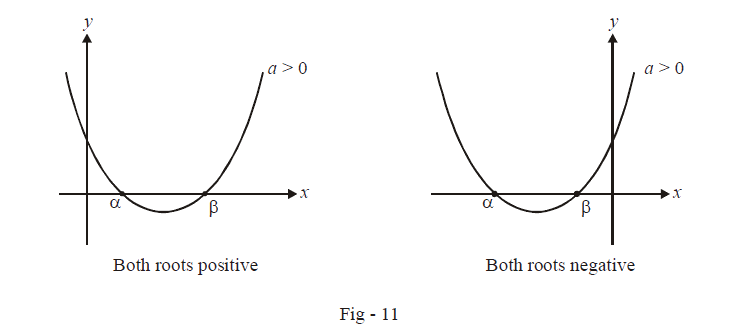Hence, the constraints are:

\fbox{\(\begin{align}&{b^2} - 4ac \ge 0\\\,\,\,\,\,\,\,\,\,&\qquad\;\;\frac{c}{a} > 0\end{align}}\)

(B)    $$\fbox{Roots are of opposite sign}$$

For real roots, $$D \ge 0.$$  For roots of opposite sign, $$\alpha \beta < 0.$$ However, notice that $$\alpha \beta < 0$$ ensures that  $$D > 0$$  and hence writing the first constraint is unnecessary (Why?).Therefore all we require is  $$\alpha \beta < 0.$$

The graphs below illustrate this case.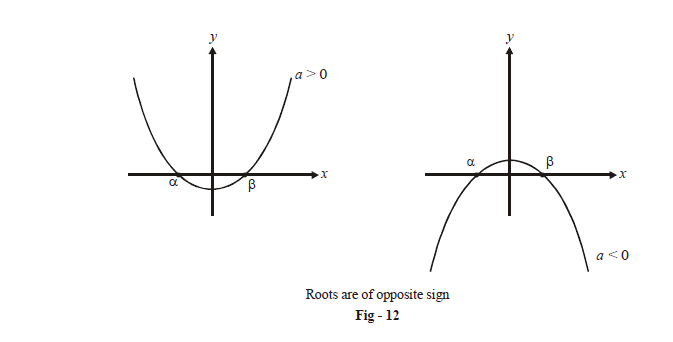The required constraint is:

$$\boxed{\frac{c}{a}< 0}$$

(C)  $$\fbox{\({\rm{Both}}\,{\rm{roots}}\,{\rm{are}}\,{\rm{positive}}$$}\)

If you have followed the previous two cases properly, this case should be straight forward. We first of all require $$D \ge 0.$$  Since both the roots are positive, $$\alpha \beta > 0\,{\rm{and}}\,\alpha + \beta > 0.$$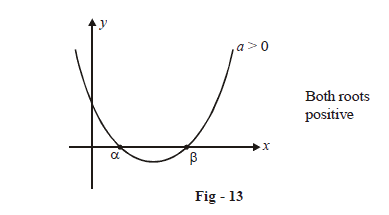\fbox{\(\begin{align}&{b^2}\,\, - 4ac \ge \,0\\&\,\,\,\,\,\,\,\frac{{ - b}}{a} > \,0\\&\,\,\,\,\,\,\,\,\,\frac{c}{a} > \,0\end{align}}\)

(D) $$\fbox{\({\rm{Both}}\,{\rm{roots}}\,{\rm{are}}\,{\rm{negative}}$$}\)

This is similar to the previous case: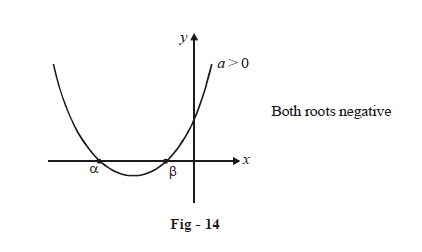\fbox{\(\begin{align}{{b}^{2}}\,\,-4ac\ge \,0 \\ \,\,\,\,\,\,\,\frac{-b}{a}<\,0 \\ \,\,\,\,\,\,\,\,\,\frac{c}{a}>\,0 \\ \end{align}}\)

(E)   $$\fbox{\({\rm{Roots}}\,{\rm{lie}}\,{\rm{on}}\,{\rm{either}}\,{\rm{side}}\,{\rm{of}}\,k$$}\)

This means that  $$\alpha < k,\,\,\beta > k$$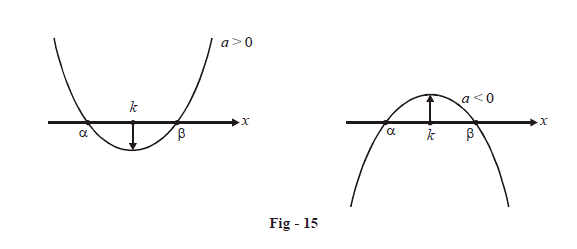We see that if $$a > 0,\,f\left( k \right) < 0$$ and if  $$a < 0,f\left( k \right) > 0$$ or to put concisely,  $$af\left( k \right) < 0.$$  This represents a necessary and also sufficient constraint for our requirement (verify that this condition alone is sufficient):

$$\fbox{\(af\left( k \right) < 0$$}\)

(F)    $$\fbox{\({\rm{Both}}\,{\rm{the}}\,{\rm{roots}}\,{\rm{are}}\,{\rm{less}}\,{\rm{than}}\,k$$}\)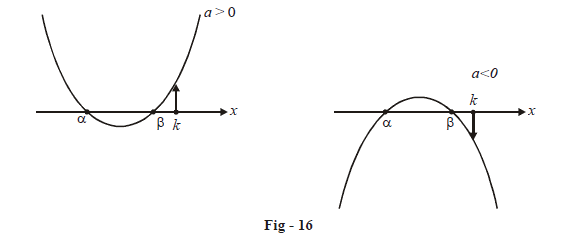We see that for the left hand graph  $$\left( {a > 0} \right),f\left( k \right) > 0$$  and for the right hand graph  $$\left( {a < 0} \right),f\left( k \right) < 0.$$  We can say this concisely as  $$af\left( k \right) > 0.$$ We also require $$D \ge 0$$  and since both the roots are less than k, $$\alpha + \beta < 2k$$

Hence, the constraints are:

\fbox{\(\begin{align}&D \ge 0\\&af\left( k \right) > 0\\ &- \frac{b}{a} < 2k\end{align}}\)

(G)   $$\fbox{\({\rm{Both}}\,{\rm{the}}\,{\rm{roots}}\,{\rm{are}}\,{\rm{greater}}\,{\rm{than}}\,k$$}\)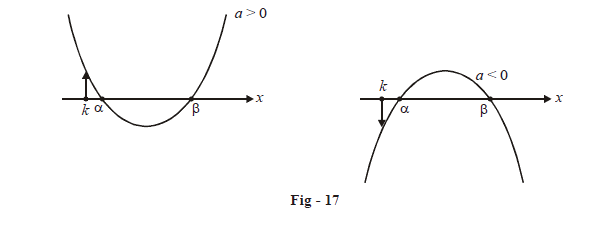Here again, we see that  $$af\left( k \right) > 0.$$  Also, $$\alpha + \beta > 2k$$

\fbox{\(\begin{align}&D \ge 0\\&af\left( k \right) > 0\\ &- \frac{b}{a} > 2k\end{align}}\)

(H) $$\fbox{\({\rm{Both}}\,{\rm{the}}\,{\rm{roots}}\,{\rm{lie}}\,{\rm{between}}\,{k_1}\,{\rm{and}}\,{k_2}$$}\)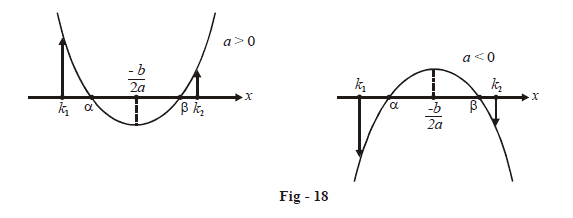First of all, $$D \ge 0$$  for real roots. Now, notice that the x-coordinate of the vertex of the parabola lies between $${k_1}\,\,and\,\,{k_2}$$. Also, whether a is positive or negative, notice that $$af({k_1}){\rm{ }}\,\,and \,\,af({k_2})$$ are  positive.

The constraints are:

\fbox{\(\begin{align}&D \ge 0\\&af\left( {{k_1}} \right) > 0\\&af\left( {{k_2}} \right) > 0\\&{k_1} < - \frac{b}{{2a}} < {k_2}\end{align}}\)

(I)  $$\fbox{\({\rm{Exactly}}\,{\rm{one}}\,{\rm{root}}\,{\rm{lies}}\,{\rm{between}}\,{k_1}\,{\rm{and}}\,{k_2}$$}\)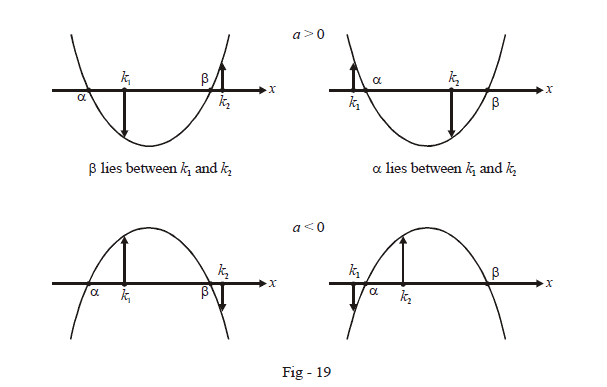In all the four possible cases, $$f\left( {{k_1}} \right){\rm{and}}\,f\left( {{k_2}} \right)$$ are of opposite sign. We can write this as $$f\left( {{k_1}} \right)f\left( {{k_2}} \right) < 0$$  . Try to see that once we write this constraint, the basic constraint  $$D \ge 0$$  becomes redundant (Why? Because  $$f (k_1)$$  and $$f (k_2)$$ can be of opposite sign only if the graph crosses the axis; this means that writing  $$f\left( {{k_1}} \right) \cdot f\left( {{k_2}} \right) < 0$$  automatically implies that  $$f\left( x \right)$$  will have real roots).

The required constraint is.

$$\fbox{\(f\left( {{k_1}} \right) \cdot f\left( {{k_2}} \right) < 0$$}\)

(J)  $$\fbox{\({k_1}\,{\rm{and}}\,{k_2}\,{\rm{lie}}\,{\rm{between}}\,{\rm{the}}\,{\rm{roots}}$$}\)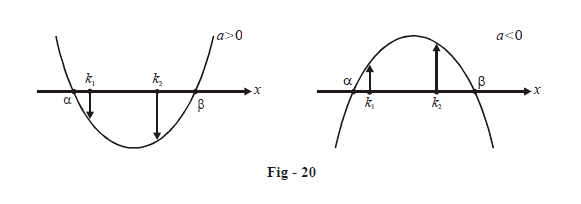Here, we see that for both cases $$af\left( {{k_1}} \right){\rm{and}}\,af\left( {{k_2}} \right)$$  will be negative. Notice again that this represents a sufficient condition.

\fbox{\(\begin{align}&af\left( {{k_1}} \right) < 0\\&af\left( {{k_2}} \right) < 0\end{align}}\)

Learn from the best math teachers and top your exams

• Live one on one classroom and doubt clearing
• Practice worksheets in and after class for conceptual clarity
• Personalized curriculum to keep up with school